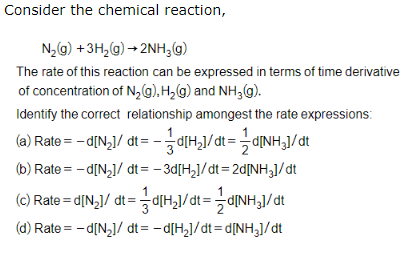For a given reaction, presence of catalyst reduces the energy of activation by 2 kcal at 27$°$C. The rate of reaction will be increased by:

1. 20 times

2. 14 times

3. 28 times

4. 2 times

High Yielding Test Series + Question Bank - NEET 2020

Difficulty Level:

What fraction of a reactant showing first order remains after 40 minute if t1/2 is 20 minute?

(a) 1/4

(b) 1/2

(c) 1/8

(d) 1/6

High Yielding Test Series + Question Bank - NEET 2020

Difficulty Level:

Rate equation for a second order reaction is:

(a) K = (2.303/t) log {a/(a-x)}

(b) K = (1/t) log {a/(a-x)}

(c) K = (1/t) log {a/a(a-x)}

(d) K = (1/t2) log {a/(a-x)}

High Yielding Test Series + Question Bank - NEET 2020

Difficulty Level:

For the reaction 2NO2 + F2 → 2NO2F, following

mechanism has been provided,

NO2 + F2   $\stackrel{slow}{\to }$  NO2F+F

NO2 + F   $\stackrel{fast}{\to }$ NO2F

Thus, rate expression of the above

reaction can be written as:

(a) r = K[NO2]2[F2]

(b) r = K[NO2 ][F2]

(c) r = K[NO2]

(d) r = K[F2]

High Yielding Test Series + Question Bank - NEET 2020

Difficulty Level:

For the reaction:

[Cu(NH3)4]2+ + H2O$⇌$[Cu(NH3)3H2O]2+ + NH3

the net rate of reaction at any time is given by, net rate =

2.0x10-4 [Cu(NH3)4]2+[H2O] - 3.0x105 [Cu(NH3 )3 H20]2+[NH3]

Then correct statement is/are :

(a) rate constant for forward reaction = 2 x 10-4

(b) rate constant for backward reaction = 3 x 105

(c) equilibrium constant for the reaction = 6.6 x 10-10

(d) all of the above

High Yielding Test Series + Question Bank - NEET 2020

Difficulty Level:

Rate constant of reaction can be expressed by Arrhenius equation as,

$K=A{e}^{-{E}_{a}}{RT}}$

In this equation, ${\mathrm{E}}_{\mathrm{a}}$ represents:

(a) the energy above which all the colliding molecules will react

(b) the energy below which colliding molecules will not react

(c) the total energy of the reacting molecules at a temperature, T

(d) the fraction of molecules with energy greater than the activation energy of the reaction

High Yielding Test Series + Question Bank - NEET 2020

Difficulty Level:High Yielding Test Series + Question Bank - NEET 2020

Difficulty Level:

For a first order reaction A$\to$ Product, the initial concentration of A is 0.1 M and after 40 minute it becomes 0.025 M. Calculate the rate of reaction at reactant concentration of 0.01M:

(a) 3.47x10-4 M min-1

(b) 3.47x10-5 M min-1

(c) 1.735 x 10-6 M min-1

(d) 1.735 x10-4 M min-1

High Yielding Test Series + Question Bank - NEET 2020

Difficulty Level:

Select the intermediate in the following reaction mechanism:

O3(g) $⇌$ O2(g) +O(g)

O(g) +O3(g) $\to$ 2O2(g)

(a) O3(g)

(b) O(g)

(c) O2(g)

(d) none of these

High Yielding Test Series + Question Bank - NEET 2020

Difficulty Level:

A reactant with initial concentration 1.386 mol litre-1 showing first order change takes 40 minute to become half. If it shows zero order change taking 20 minute to becomes half under the similar conditions, the ratio, K1/K0 for first order and zero order kinetics will be:

(a) 0.5 mol-1 litre

(b) 1.0 mol/litre

(c) 1.5 mol/litre

(d) 2.0 mol-1 litre